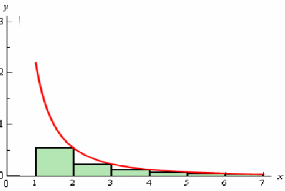## Overestimates and Underestimates of Integrals

We can estimate an integral using the trapezium or the mid – ordinate rule. Sometimes however it is desirable to find underestimates and overestimates for an integral. The closer these are, the more accurately can the value for the integral be found and we can also quantify the error for the estimate. The estimate below shows the graph ofWe can find an underestimate forby finding the minimum value of y on each of the intervalsand adding the areas of the rectangles on each interval with these heights.Ifis the actual value of the integral, thenSimilarly an overestimate for I can be found by finding the maximum value ofon each of the intervals and adding the areas of the triangles on each interval with these heights.Hence